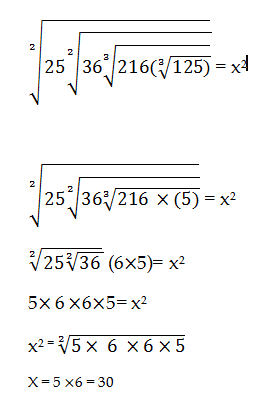# RRB ALP 2018 Practice Test Papers | Arithmetic Questions (Day-66)

Dear Aspirants, Here we have given the Important RRB ALP & Technicians Exam 2018 Practice Test Papers. Candidates those who are preparing for RRB ALP 2018 can practice these Arithmetic Questions to get more confidence to Crack RRB 2018 Examination.

[WpProQuiz 2912]

Click “Start Quiz” to attend these Questions and view Solutions

1) Anita and Aishu have some chocolate in ratio of 4:3. Anita gives 3 chocolate to Aishu then the ratio will become equal. How many chocolates Anita have initially?

a) 22

b) 24

c) 26

d) 30

2) Average age of a family consists of 4 members was 42 before 3 years. Now the family has 5 members so as the average age is increased by 1. What is the age of new member?

a) 30

b) 25

c) 35

d) 40

3) 225÷15÷5÷3 =?

a) 100

b) 20

c) 1

d) 10

4) A fraction is added with its reciprocal gives five times of same fraction. Find the fractional value

a) 1/2

b) 1/3

c) 2/3

d) 4/5

5) 95÷5 × 85÷ 5 + 30%of 560 + 32 =?

a) 100

b) 250

c) 500

d) 1000

6) Find the missing number

67.5 22.5 9 4.5 3 ? 6

a) 2

b) 1.5

c) 3

d) 1

7) A student got 56% of marks in his examination out of total marks is 500. What the total marks secured by him.

a) 140

b) 160

c) 280

d) 200

8) 8 ½ + 2 ¾ + 3 ½ = x + 4 ½ + 6 ¾ =    ?

a) 1 ½

b) 2 ½

c) 3 ½

d) 4 ½

9)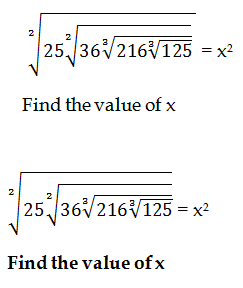10). Let’s chat on Hangouts!
897 + 13 – 400 = ?

a) 320
b) 420
c) 410
d) 100

No. of chocolates = 4x, 3x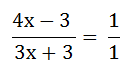4x – 3 = 3x + 3

4x – 3x = 3 +3 = 6

X = 6

Anita has 24 chocolates initially

Sum of their ages before 3 years = 42×4 = 168

Sum of their ages in present = 168 + (4×3) = 168+12 = 180

Sum of their ages including new person = 5×43 = 215

Age of New member = 215 – 180 = 35

225 × 1/15 × 1/5 × 1/ 3 =1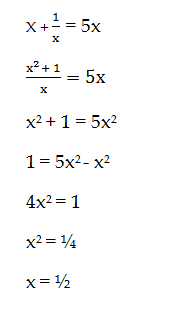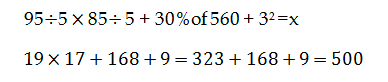67.5 ÷3 = 22.5

22.5 ÷ 2.5 = 9

9 ÷ 2 = 4.5

4.5 ÷ 1.5 = 3

3÷1 = 3

3 ÷ 0.5 = 6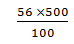= 280

8 + 2 + 3 – 4 – 6 (½ + ¾ + ½ – ½ – ¾)

= 3 ½